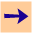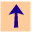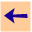8 Built-in predicates

# 8 Built-in predicates

There are a few built-in predicates. These cannot be redefined. The following arithmetic predicates are predefined:
• X < Y, where X and Y must be ground arithmetic expressions when called, is true if the value of X is less than the value of Y.
• X > Y, where X and Y must be ground arithmetic expressions when called, is true if the value of X is greater than the value of Y.
• X =< Y, where X and Y must be ground arithmetic expressions when called, is true if the value of X is less than or equal to the value of Y.
• X >= Y, where X and Y must be ground arithmetic expressions when called, is true if the value of X is greater than or equal to the value of Y.
• X =\= Y, where X and Y must be ground arithmetic expressions when called, is true if the value of X is different to the value of Y.
• V is E where E must be a ground arithmetic expression when called. This is true if expression E evaluates to V.
• number(N), where N is ground is true if N is a number.
Arithmetic expressions can either be numbers or of the form (E1+E2), (E1-E2), (E1*E2), (E1/E2), sin(E), log(E), etc.

Example. The following shows a simple use of is and >. It shows how we can use variables in the expressions, as long as they are bound before (to the left) they are used.

```cilog: ask X is 3+4*5 & X*2 > 11*3.
Answer: 23 is 3+4*5 & 23*2>11*3.
```

The restriction that the arithmetic expressions must be ground when called is severe, and probably won't be fixed in future implementations.

There is one non-arithmetic comparison:

• `X \= Y` true if X and Y denote different objects under the unique names assumption.
X and Y must be bound enough to determine whether they can be unified. (Delaying is not implemented). This may be fixed in future versions.8 Built-in predicates